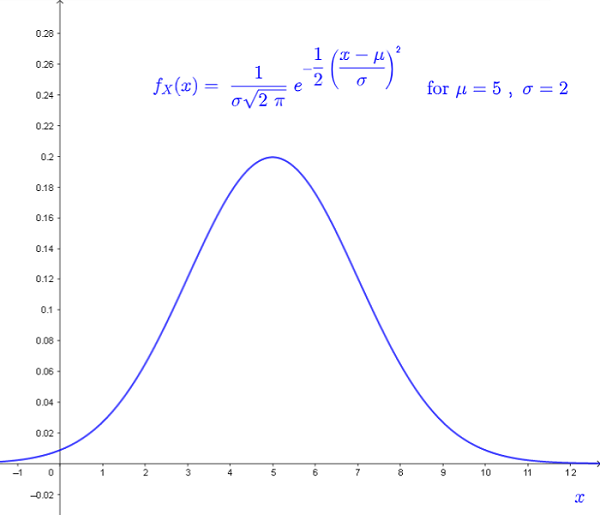# The Error Function Erf(x) and Normal Distribution

     

## Error Function

The error function $\text{Erf} \; (x)$ is defined by the integral  $\displaystyle \text{Erf} \; (x) = \dfrac{2}{\sqrt{\pi}} \int_0^{x} \; e^{-t^2} \; dt$ Computer programs, in different languages, may easily be developed to calculate the $\text{Erf} \; (x)$.
A table of values of $\text{Erf} (x)$ in the interval $x \in [-3 \; , \; 3]$ was created using Google sheets is presented below along with its graph. (you might need to scroll down).

From the definition and the graph, we can say that $\text{Erf} \; (x)$ is an odd function and therefore
$\qquad \text{Erf} \; (-x) = -\text{Erf} \; (x)$

## Normal Distribution Cumulative Function

The normal density function of a random variabe $X$ with mean $\mu$ and standard deviation $\sigma$ is given by    .
$f_{X}(x) = \dfrac{1}{\sigma \sqrt{2 \; \pi }} \; e^{-\frac{1}{2} \left(\dfrac{x -\mu}{\sigma} \right)^2 } \qquad (I)$
whise graph is shown below.The cumulative distribution function of $f_{X}(x)$ is given by
$F_{X}(x,\mu,\sigma) = \int_{-\infty}^{x} f_{X}(t) dt$
When $f_{X}(t)$ is substituted by $f_{X}$ defined in $(I)$, we have $\displaystyle F_{X}(x,\mu,\sigma) = \dfrac{1}{\sigma \sqrt{2 \; \pi }} \int_{-\infty}^{x} \; e^{-\frac{1}{2} \left(\dfrac{t -\mu}{\sigma} \right)^2 } dt \qquad (II)$
$F_{X}(x,\mu,\sigma)$ is used to calculate the probabilities as follows
$\qquad P( X \lt x) = F_{X}(x,\mu,\sigma)$
$\qquad P( b \le X \le a) = F_{X}(a) - F_{X}(b)$

## Relationship between $F_{X}(x,\mu,\sigma)$ and $\text{Erf} \; (x)$

We now develop the relationship between the cumulative distribution function for a normal distribution defined above and given by $F_{X}(x,\mu,\sigma) = \dfrac{1}{\sigma \sqrt{2 \; \pi }} \int_{-\infty}^{x} \; e^{-\frac{1}{2} \left(\dfrac{t -\mu}{\sigma} \right)^2 } dt \qquad (III)$ and the error function defined by $\displaystyle \text{Erf} \; (x) = \dfrac{2}{\sqrt{\pi}} \int_0^{x} \; e^{-t^2} \; dt \qquad (IV)$

Let $z = \dfrac{t-\mu}{\sigma \sqrt 2}$ and therefore $\dfrac{dz}{dt} = \dfrac{1}{\sigma \sqrt 2}$ or $dz = \dfrac{1}{\sigma \sqrt 2} dt$
Substitute the above in $(III)$ and write $\displaystyle F_{X}(x,\mu,\sigma) = \dfrac{1}{ \sqrt{\pi} } \int_{-\infty}^{\dfrac{x-\mu}{\sigma \sqrt 2}} \; e^{-z^2 } dz$
Split the interval of integration and write $F_{X}(x,\mu,\sigma)$ as follows $\displaystyle F_{X}(x,\mu,\sigma) = \dfrac{1}{ \sqrt{\pi} } \left( \int_{-\infty}^{0} \; e^{-z^2 } dz + \int_{0}^{\dfrac{x-\mu}{\sigma \sqrt 2}} \; e^{-z^2 } dz \right) \qquad (V)$

We now use the Gaussian integral Gaussian integral which is given by $\int_{-\infty}^{+\infty} e^{-x^2} dx = \sqrt {\pi}$ and because $e^{-x^2}$ is an even function, we have $\int_{-\infty}^{0} e^{-x^2} dx = \int_{0}^{\infty} e^{-x^2} dx$ Use the Gaussian integral to write $\int_{-\infty}^{0} e^{-x^2} dx = \int_{0}^{\infty} e^{-x^2} dx = \dfrac{\sqrt {\pi}}{2}$ Substitute in $\qquad (V)$ and write $\displaystyle F_{X}(x,\mu,\sigma) = \dfrac{1}{ \sqrt{\pi} } \left( \dfrac{\sqrt {\pi}}{2} + \int_{0}^{\dfrac{x-\mu}{\sigma \sqrt 2}} \; e^{-z^2 } dz \right) \qquad (V)$ Using $(IV)$ , we write $\displaystyle \int_0^{\left(\dfrac{x - \mu}{\sigma\sqrt 2}\right)} \; e^{-t^2} \; dt = \dfrac{\sqrt{\pi}}{2} \text{Erf} \; \left(\dfrac{x - \mu}{\sigma\sqrt 2}\right)$ which we substitute in $(V)$ above to write

$\displaystyle F_{X}(x,\mu,\sigma) = \dfrac{1}{ \sqrt{\pi} } \left( \dfrac{\sqrt {\pi}}{2} + \dfrac{\sqrt{\pi}}{2} \text{Erf} \; \left(\dfrac{x - \mu}{\sigma\sqrt 2}\right) \right) \qquad (V)$ Simplify and write the relationship between between $F_{X}(x,\mu,\sigma)$ and $\text{Erf} \; (x)$ as follows: $\boxed F_{X}(x,\mu,\sigma) = \dfrac{1}{2 } \left( 1 + \text{Erf} \; \left(\dfrac{x - \mu}{\sigma\sqrt 2}\right) \right)}$ Hence the normal distribution cumulative function $F_{X}(x,\mu,\sigma)$ may be calculated using the error function $\text{Erf} (x)$.